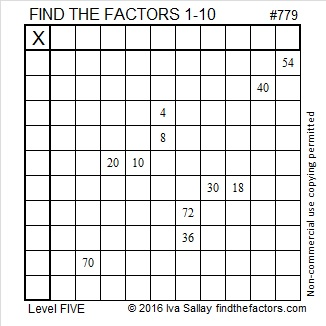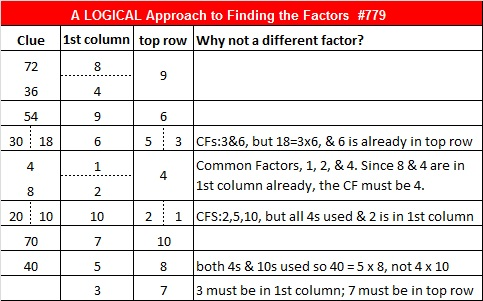# Can You See How 779’s Factor Pairs Are Hiding in Some Pythagorean Triples?

• 779 is a composite number.
• Prime factorization: 779 = 19 x 41
• The exponents in the prime factorization are 1 and 1. Adding one to each and multiplying we get (1 + 1)(1 + 1) = 2 x 2 = 4. Therefore 779 has exactly 4 factors.
• Factors of 779: 1, 19, 41, 779
• Factor pairs: 779 = 1 x 779 or 19 x 41
• 779 has no square factors that allow its square root to be simplified. √779 ≈ 27.91057.Those factor pairs are hiding in some Pythagorean triples. Scroll down to read how, but first here’s today’s puzzle:Print the puzzles or type the solution on this excel file: 10-factors-2016

————————————–

And now some Pythagorean triple number theory using 779 as an example:

The factors of 779 are very well hidden in five Pythagorean triples that contain the number 779. Here’s how: 779 has two factor pairs:  19 x 41 and 1 x 779. Those factor pairs show up in some way in each of the calculations for these 779 containing Pythagorean triples:

1. 171-760-779 which is 19 times each number in 9-40-41.
2. 779-303420-303421, a primitive calculated from 779(1); (779² – 1²)/2; (779² + 1²)/2.
3. 779-7380-7421 which is 41 times each number in 19-180-181.
4. 779-15960-15979 which is 19 times each number in 41-840-841.
5. 660-779-1021, a primitive calculated from (41² – 19²)/2; 19(41); (41² + 19²)/2.

Being able to find whole numbers that satisfy the equation a² + b² = c² is one reason why finding factors of a number is so worth it. ANY factor pair for numbers greater than 2 will produce at least one Pythagorean triple that satisfies a² + b² = c². The more factor pairs a number has, the more Pythagorean triples will exist that contain that number. 779 has only two factor pairs so there are a modest number of 779 containing Pythagorean triples. All of its factors are odd so it was quite easy to find all of the triples. Here’s a brief explanation on how each triple was found:

1. 799 has one prime factor that has a remainder of 1 when divided by 4. That prime factor, 41, is therefore the hypotenuse of a primitive Pythagorean triple. When the Pythagorean triple is multiplied by the other half of 41’s factor pair, 19, we get a Pythagorean triple in which 779 is the hypotenuse.
2. Every odd number greater than 1 is the short leg of a primitive Pythagorean triple. To find that primitive for a different odd number, simply substitute the desired odd number in the calculation in place of 779.
3. Because every odd number greater than 1 is the short leg of a primitive Pythagorean triple, 19(1); (19² – 1²)/2; (19² + 1²)/2 generates the primitive triple (19-180-181). Multiplying each number in that triple by the other half of 19‘s factor pair, 41, produces a triple with 779 as the short leg.
4. Because every odd number greater than 1 is the short leg of a primitive Pythagorean triple, 41(1); (41² – 1²)/2; (41² + 1²)/2 generates the primitive triple (41-840-841). Multiplying each number in that triple by the other half of 41‘s factor pair, 19, produces a triple with 779 as the short leg.
5. Since factor pair 19 and 41 have no common prime factors, the formula (41² – 19²)/2; 19(41); (41² + 19²)/2 produces another primitive triple 660-779-1021. If they did have common factors, the factor pair would still produce a triple, but it would not be a primitive one.

Here’s some other interesting facts about the number 779:

779 is the sum of eleven consecutive prime numbers:

47 + 53 + 59 + 61 + 67 + 71 + 73 + 79 + 83 + 89 + 97 = 779.

779 can also be written as the sum of three squares six different ways:

• 27² + 7² + 1² = 779
• 27² + 5² + 5² = 779
• 23² + 15² + 5² = 779
• 23² + 13² + 9² = 779
• 21² + 17² + 7² = 779
• 21² + 13² + 13² = 779

Finally, the table below shows some logical steps that could be used to solve Puzzle #779: